Command Reference : Matrix Language Reference

 @qr
QR decomposition of matrix.
Syntax: @qr(M, R[, P])
M: matrix
R: matrix
P: (optional) matrix
Return: matrix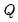Decomposes an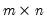matrix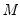into an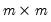orthogonal matrixand anupper triangular matrix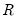such that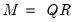, where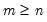.
If permutation matrix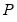is provided, the decomposition producesandsuch that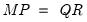.
Examples
matrix m1 = @mnrnd(7, 5)
matrix r
matrix q = @qr(m1, r)
generates a random matrix M1, then decomposes it into the orthogonal matrix Q, and the upper triangular matrix R.
The following illustrate the properties of the decomposition:
sym i1 = @inner(q)
matrix m2 = q * r
where I1 is the identity matrix, and M2 is equal to M1.
Cross-references